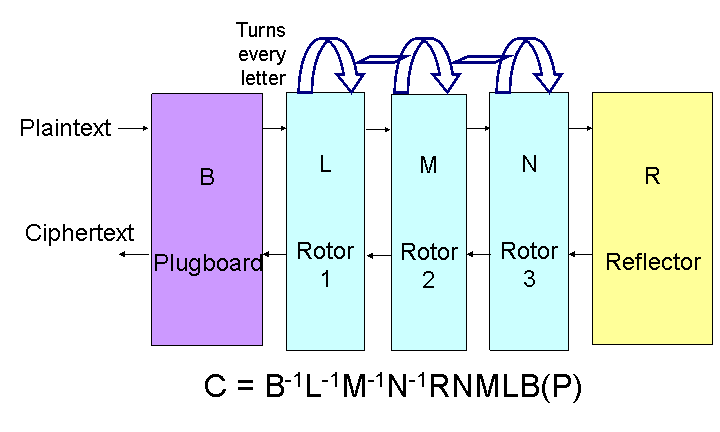University of Virginia, Department of Computer Science CS588: Cryptography, Spring 2005

Notes: Tuesday 1 February 2005
Assignments Due

Notes

How many women should we expect the Iraqi national assembly to have?

```(define number-of-parties 111)
(define number-of-assembly-members 275)

(define (max a b) (if (> a b) a b))
(define (factorial n) (if (= n 0) 1 (* n (factorial (- n 1)))))
(define (choose n k)
(/ (factorial n) (* (factorial k) (factorial (- n k)))))

(define (binomial-probability n k p)
(* (choose n k) (expt p k) (expt (- 1 p) (- n k))))

(define (expected-parties seats)
(exact->inexact (* number-of-parties
(binomial-probability number-of-assembly-members
seats (/ 1 number-of-parties)))))

(define (expected-party-count n)
(if (< n 0) 0
(+ (expected-parties n) (expected-party-count (- n 1)))))

(define (expected-women n)
(if (< n 0) 0
(+ (* (floor (/ n 3)) (expected-parties n))
(expected-women (- n 1)))))
```Enigma Schematic

How big is the keyspace for the Enigma machine?

What is an involution?

Why must the reflector be an involution?

Rejewski's Theorem: The composition of two permutations that are involutions consists of pairs of cycles of the same length.